Preschool Shapes Tracing – Heart, Star, Circle, Square, Triangle, Pentagon, Hexagon, Octagon, Oval, Rectangle, Diamond, Heptagon, Nonagon, Decagon – Fourteen Worksheets

Kindergarten Worksheets

### Preschool Worksheets

Numbers 1 – 10

Numbers 1 – 15

Number Tracing Worksheets

Shape Tracing Worksheets

Picture Tracing Worksheets

Tracing Worksheets

Shapes

Geometry

Shape Names – Shape Labels

Pre-Writing Worksheets

Preschool Shapes Tracing – 5 Worksheets

Preschool Shapes Worksheet

Preschool shapes recognition practice worksheet – Identify Shapes – Heart, Star, Circle, Square, Triangle, Pentagon, Hexagon, Octagon, Oval, Rectangle, and Diamond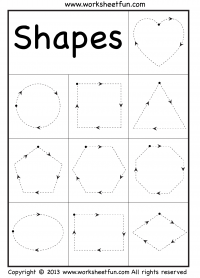Heart, Star, Circle, Square, Triangle, Pentagon, Hexagon, Octagon, Oval, Rectangle and Diamond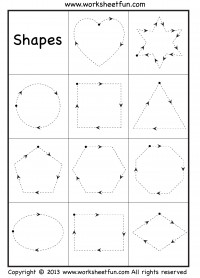Heart, Star, Circle, Square, Triangle, Pentagon, Hexagon, Octagon, Oval, Rectangle and Diamond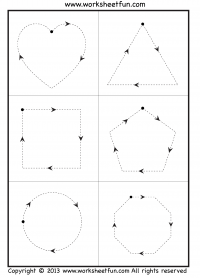Heart, Circle, Square, Triangle, Pentagon, Octagon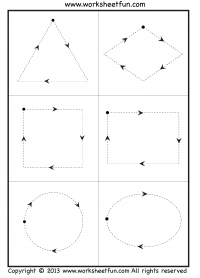Circle, Square, Triangle, Oval, Rectangle, and Diamond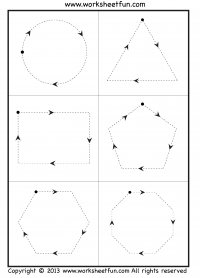Circle, Triangle, Pentagon, Hexagon, Octagon, Rectangle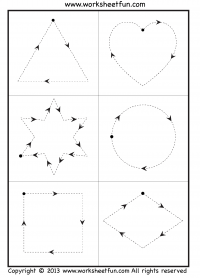Heart, Star, Circle, Square, Triangle, and Diamond

Basic Shape Names – Geometric Shape Name Labels – 2D Shapes and Labels – Shapes Names -Shapes with Labels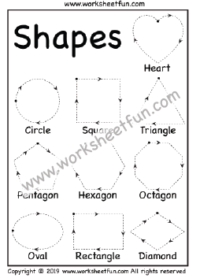Basic Shape Names – Geometric Shape Name Labels – 2D Shapes and Labels – Shapes Names -Shapes with Labels

Heart, Circle, Square, Triangle, Pentagon, Hexagon, Octagon, Oval, Rectangle and Diamond – Worksheet 7here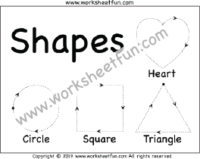Basic Shape Names – Geometric Shape Name Labels – 2D Shapes and Labels – Shapes Names -Shapes with Labels

Heart, Circle, Square, Triangle – Worksheet 8 –here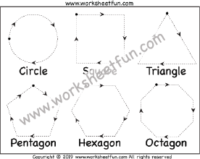Basic Shape Names – Geometric Shape Name Labels – 2D Shapes and Labels – Shapes Names -Shapes with Labels

Circle, Square, Triangle, Pentagon, Hexagon, Octagon – Worksheet 9 here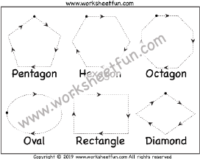Basic Shape Names – Geometric Shape Name Labels – 2D Shapes and Labels – Shapes Names -Shapes with Labels

Pentagon, Hexagon, Octagon, Oval, Rectangle, and Diamond – Worksheet 10- here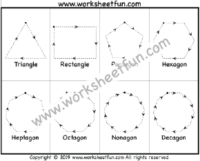Triangle, Rectangle, Pentagon, Hexagon, Heptagon, Octagon, Nonagon, DecagonWorksheet 11 –here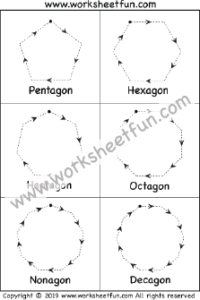Pentagon, Hexagon, Heptagon, Octagon, Nonagon, DecagonWorksheet 12 –here

Practice Picture Tracing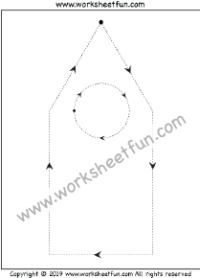Circle, Triangle, and Rectangle – Worksheet 13 – here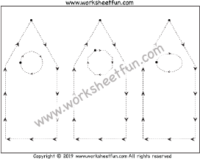Circle, Octagon, Oval, Triangle, and Rectangle – Worksheet 14 – here

Shapes

Shape Names – Shape Labels

Geometry

Letter Tracing Worksheets

Number Tracing Worksheets

Shape Tracing Worksheets

Picture Tracing Worksheets

Tracing Worksheets

### Preschool Worksheets